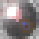2647 visitas
small update: added ability to setup stage 2 length period.
```study(title="[RS]Vector Flow Channel V1", shorttitle="[RS]VFC.V1", overlay=true)
decay = atr(10)*0.005
stage1 = input(5)
stage2 = input(10)
stage3 = input(20)
stage1length = input(100)
stage2length = stage1length + input(100)
pretopvector01 = nz(topvector01, close)
topvector01 = close >= pretopvector01 ? close : pretopvector01 - (decay * topvector01finalcounter)
topvector01counter = n - valuewhen(close >= topvector01, n, 0)
topvector01finalcounter = topvector01counter <= stage1length ? stage1 : topvector01counter <= stage2length ? stage2 : stage3

prebotvector01 = nz(botvector01, close)
botvector01 = close <= prebotvector01 ? close : prebotvector01 + (decay * botvector01finalcounter)
botvector01counter = n - valuewhen(close <= botvector01, n, 0)
botvector01finalcounter = botvector01counter <= stage1length ? stage1 : botvector01counter <= stage2length ? stage2 : stage3

pt = plot(topvector01, color=(topvector01 == close ? na : black))
pb = plot(botvector01, color=(botvector01 == close ? na : black))
fill(pt, pb, color=blue, transp=90)
```

## Comentariosyes this is based on linear regression
ResponderRicardoSantos
Thanks, this is actually very useful for me. Much more than just chart art.
ResponderRicardoSantos
no offense, but how is it based on regression? there is no regression computation in the code.
Responderisomorph
my apologies, poorly used term :s
it would likely make more sense as mean regression.
ResponderRicardoSantos
ok no worries. could you please explain a little more about the origin of the algorithm? is this something you found on some site like stockcharts.com or investopedia? or is it your idea and if so, what is the main rationale behind it?

i ask because i am puzzled by this notion of using atr as a decay factor. in other words, why? why think of atr as 'decay'?
Responderisomorph
not at all, is mostly inspired by sar and gann fan's visual appearance, the rationale is the perception of price decay over time.

the atr was just a means to a end, it could be very well an input, pip or other unit of measurement.
ResponderRicardoSantos
ok thanks. in your testing, what did you find out regarding the usefulness of study VFC for TFs < daily?
Responderdave.seremek
i suppose i could say some crazy stuff like "imagine that price is like a leaf in the river of time flowing with the current and being dragged by the wind" to sound smart lol...
but in truth its a visual perception trick caused by the brain. support and resistance is as a reactionary effect of human psychology nature?
i suppose im really bad at explaining stuff lol
Responder